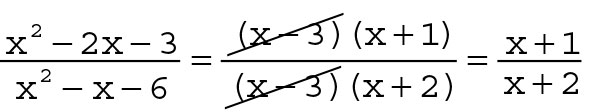##### Pre-Calculus For Dummies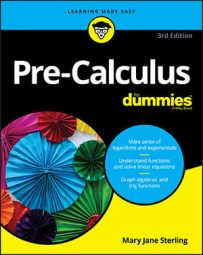## Figure Out What the Problem Is Asking

Often, you’ll find that reading comprehension and the ability to work with multiple parts that comprise a whole is an underlying property of a math problem. That’s okay — that’s also what life is all about!! When faced with a math problem, start by reading the whole problem or all the directions to the problem. Look for the question inside the question. Keep your eyes peeled for words like solve, simplify, find, and prove, all of which are common buzz words in any math book. Don’t begin working on a problem until you’re certain of what it wants you to do.

For example, take a look at this problem:

The length of a rectangular garden is 24 feet longer than the garden’s width. If you add 2 feet to both the width and the length, the area of the garden is 432 square feet. How long is the new, bigger garden?
If you miss any of the important information, you may start to solve the problem to figure out how wide the garden is. Or you may find the length but miss the fact that you’re supposed to find out how long it is with 2 feet added to it. Look before you leap!

Underlining key words and information in the question is often helpful. This can’t be stressed enough. Highlighting important words and pieces of information solidifies them in your brain so that as you work, you can redirect your focus if it veers off-track. When presented with a word problem, for example, first turn the words into an algebraic equation. If you’re lucky and are given the algebraic equation from the get-go, you can move on to the next step, which is to create a visual image of the situation at hand.

And, if you’re wondering what the answer to the example problem is, you’ll find out as you read further.

## Draw Pictures (the More the Better)

Your brain is like a movie screen in your skull, and you’ll have an easier time working problems if you project what you see onto a piece of paper. When you visualize math problems, you’re more apt to comprehend them. Draw pictures that correspond to a problem and label all the parts so you have a visual image to follow that allows you to attach mathematical symbols to physical structures. This process works the conceptual part of your brain and helps you remember important concepts. As such, you’ll be less likely to miss steps or get disorganized.

If the question is talking about a triangle, for instance, draw a triangle; if it mentions a rectangular garden filled with daffodils for 30 percent of its space, draw that. In fact, every time a problem changes and new information is presented, your picture should change, too.

If you were asked to solve the rectangular garden problem from the previous section, you’d start by drawing two rectangles: one for the old, smaller garden and another for the bigger one. Putting pen or pencil to the paper starts you on the way to a solution.

When you know and can picture what you must find, you can plan your attack from there, interpreting the problem mathematically and coming up with the equations that you’ll be working with to find the answer:
1. Start by writing a statement. In the garden problem from the last two sections, you’re looking for the length and width of a garden after it has been made bigger. With those in mind, define some variables:
• Let x = the garden’s width now.
• Let y = the garden’s length now.
2. Now add those variables to the rectangle you drew of the old garden.
3. But you know that the length is 24 feet greater than the width, so you can rewrite the variable y in terms of the variable x so that y = x + 24.
4. You know that the new garden has had 2 feet added to both its width and length, so you can modify your equations:
• Let x + 2 = the garden’s new width.
• Let y + 2 = x + 24 + 2 = x + 26 the garden’s new length.
5. Now add these labels to the picture of the new garden.
By planning your attack, you’ve identified the pieces of the equation that you need to solve.

## Write Down Any Formulas

If you start your attack by writing the formula needed to solve the problem, all you have to do from there is plug in what you know and then solve for the unknown. A problem always makes more sense if the formula is the first thing you write when solving. Before you can do that, though, you need to figure out which formula to use. You can usually find out by taking a close look at the words of the problem.

In the case of the garden problem from the previous sections, the formula you need is that for the area of a rectangle. The area of a rectangle is A = lw. You’re told that the area of the new rectangle is 432 square feet, and you have expressions representing the length and width, so you can replace A = lw with 432 = (x + 26)(x + 2).

As another example, if you need to solve a right triangle, you may start by writing down the Pythagorean Theorem if you know two sides and are looking for the third. For another right triangle, perhaps you’re given an angle and the hypotenuse and need to find the opposite side; in this situation, you’d start off by writing down the sine ratio.

## Show Each Step of Your Work

Yes, you’ve been hearing it forever, but your third-grade teacher was right: Showing each step of your work is vital in math. Writing each step on paper minimizes silly mistakes that you can make when you calculate in your head. It’s also a great way to keep a problem organized and clear. And it helps to have your work written down when you get interrupted by a phone call or text message — you can pick up where you left off and not have to start all over again. It may take some time to write every single step down, but it’s well worth your investment.

## Know When to Quit

Sometimes a problem has no solution. Yes, that can be an answer, too! If you’ve tried all the tricks in your bag and you haven’t found a way, consider that the problem may have no solution at all. Some common problems that may not have a solution include the following:
• Absolute-value equations

This happens when the absolute value expression is set equal to a negative number. You may not realize the number is negative, at first, if it’s represented by a variable.

• Equations with the variable under a square-root sign

If your answer has to be a real number, and complex numbers aren’t an option, then the expression under the radical may represent a negative number. Not allowed.

When a quadratic isn’t factorable and you have to resort to the quadratic formula, you may run into a negative under the radical; you can’t use that expression if you’re allowed only real answers.

• Rational equations

Rational expressions have numerators and denominators. If there’s a variable in the denominator that ends up creating a zero, then that value isn’t allowed.

• Trig equations

Trig functions have restrictions. Sines and cosines have to lie between –1 and 1. Secants and cosecants have to be greater than or equal to 1 or less than or equal to –1. A perfectly nice-looking equation may create an impossible answer.

On the other hand, you may get a solution for some problem that just doesn’t make sense. Watch out for the following situations:

• If you’re solving an equation for a measurement (like length or area) and you get a negative answer, either you made a mistake or no solution exists. Measurement problems include distance, and distance can’t be negative.
• If you’re solving an equation to find the number of things (like how many books are on a bookshelf) and you get a fraction or decimal answer, then that just doesn’t make any sense. How could you have 13.4 books on a shelf?

Even the best mathematicians make mistakes. When you hurry through calculations or work in a stressful situation, you may make mistakes more frequently. So, check your work. Usually, this process is very easy: You take your answer and plug it back into the equation or problem description to see if it really works. Making the check takes a little time, but it guarantees you got the question right, so why not do it?

For example, if you go back and solve the garden problem from earlier in this chapter by looking at the equation to be solved: 432 = (x + 26)(x + 2), you multiply the two binomials and move the 432 to the other side. Solving x2 + 28x – 380 = 0 you get x = 10 and x = –38. You disregard the x = –38, of course, and find that the original width was 10 feet. So, what was the question? It asks for the length of the new, bigger garden. The length of the new garden is found with y = x + 26. So, the length (and answer) is that the length is 36 feet. Does this check? If you use the new length or 36 and the new width of x + 2 = 12 and multiply 36 times 12 you get 432 square feet. It checks!

## Practice Plenty of Problems

You’re not born with the knowledge of how to ride a bike, play baseball, or even speak. The way you get better at challenging tasks is to practice, practice, practice. And the best way to practice math is to work the problems. You can seek harder or more complicated examples of questions that will stretch your brain and make you better at a concept the next time you see it.

Along with working along on the example problems in this book, you can take advantage of the For Dummies workbooks, which include loads of practice exercises. Check out Trigonometry Workbook For Dummies, by Mary Jane Sterling, Algebra Workbook and Algebra II Workbook For Dummies, both also by Mary Jane Sterling, and Geometry Workbook For Dummies, by Mark Ryan (all published by Wiley), to name a few.

Even a math textbook is great for practice. Why not try some (gulp!) problems that weren’t assigned, or maybe go back to an old section to review and make sure you’ve still got it? Typically, textbooks show the answers to the odd problems, so if you stick with those you can always double-check your answers. And if you get a craving for some extra practice, just search the Internet for “practice math problems” to see what you can find! For example, if you search the Internet for “practice systems of equations problems” you’ll find more than a million hits. That’s a lot of practice!

## Keep Track of the Order of Operations

Don’t fall for the trap that always is lying there by performing operations in the wrong order. For instance, 2 – 6 × 3 doesn’t become –4 × 3 = –12. You’ll reach those incorrect answers if you forget to do the multiplication first. Focus on following the order of PEMDAS every time, all the time:
Parentheses (and other grouping devices)

Exponents and roots

Multiplication and Division from left to right

Addition and Subtraction from left to right

Don’t ever go out of order, and that’s an order!

## Use Caution When Dealing with Fractions

Working with denominators can be tricky. It’s okay to write: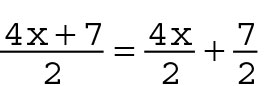But, on the other hand: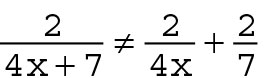Also, reducing or cancelling in fractions can be performed incorrectly. Every term in the numerator has to be divided by the same factor — the one that divides the denominator. So, it’s true that: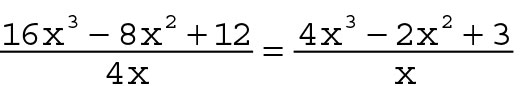because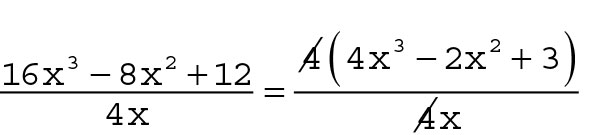But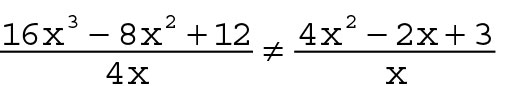, because the factor being divided out is just 4, not 4x.

And, again, it has to be the same factor that’s dividing, throughout. Can you spot the error here?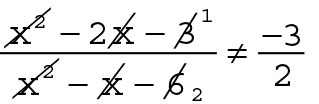Some poor soul reduced each term and the term directly above it — separately. Nope, doesn’t work that way. The correct process is to factor the trinomials and then divide by the common factor: